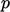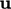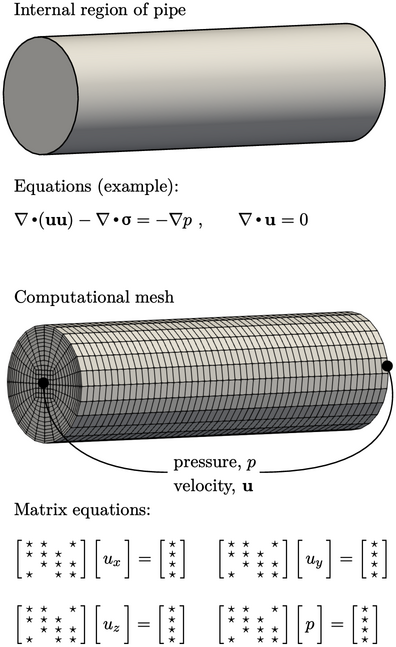## 1.1Solution overview

Let us imagine calculating ﬂuid ﬂow along a pipe with CFD. To perform the calculation ﬁrst requires a description of the problem by:

• the domain occupied by the ﬂuid, i.e. the internal region of the pipe;
• equations that represent the ﬂuid behaviour, in terms of properties such as pressureand velocity;
• conditions at the boundary of the ﬂuid domain and initially within the domain for the ﬂuid properties.

This description is represented in CFD by the following:

• a computational mesh for the ﬂuid;
• “discrete” equations and algorithms to calculateand;
• boundary and initial conditions forand.

Chapter 2 introduces the governing equations and basic models for ﬂuid motion, forces and heat. Turbulence, which commonly occurs in many ﬂows, is introduced in Chapter 6 and its standard modelling is described in Chapter 7 .

The ﬁnite volume method is presented in Chapter 3 to express equations in discrete form using its geometric representation of a computational mesh. The algorithms used to solve the matrix equations and to couple sets of equations are given in Chapter 5 .

Chapter 4 describes boundary conditions ﬁrst from a numerical perspective, i.e. how they modify the matrix equations to inﬂuence the solution. It then covers a range of conditions that represent the behaviour at boundaries in many problems.Notes on CFD: General Principles - 1.1 Solution overview